Skip to search formSkip to main content
You are currently offline. Some features of the site may not work correctly.

# Strength of a graph

Known as: Strength of a graph (graph theory)
In the branch of mathematics called graph theory, the strength of an undirected graph corresponds to the minimum ratio edges removed/components… Expand
Wikipedia

## Papers overview

Semantic Scholar uses AI to extract papers important to this topic.
2017
2017
• Discuss. Math. Graph Theory
• 2017
• Corpus ID: 7298325
Abstract New graph characteristic, the total H-irregularity strength of a graph, is introduced. Estimations on this parameter are… Expand
2017
2017
• A. Skoda
• Discret. Optim.
• 2017
• Corpus ID: 45381530
A correspondence P associates to every subset A ⊆ N a partition P(A) of A and to every game (N,v), the P-restricted game (N,vP… Expand
•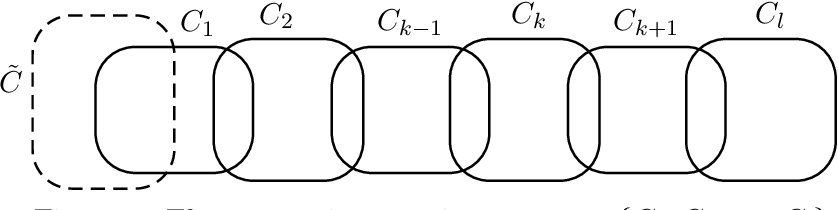•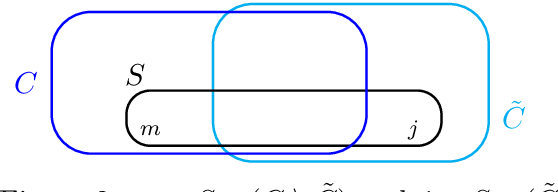•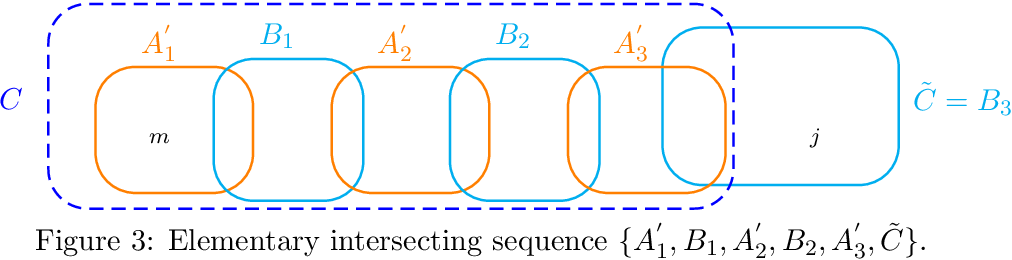•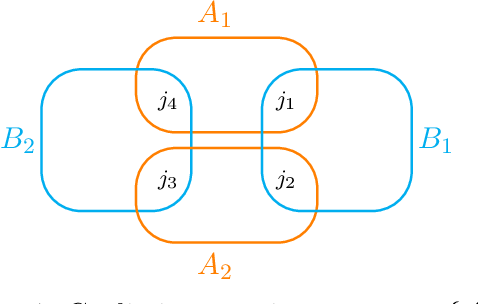•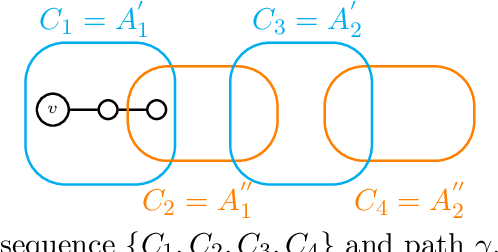2013
2013
Abstract Let G = ( V , E ) be a graph, and let c : V → { 1 , 2 , … , k } be a not necessarily proper edge colouring. The weight… Expand
2007
2007
• KyotoCGGT
• 2007
• Corpus ID: 31788058
A graph G is called super edge-magic if there exists a one-to-one mapping f from V (G ) *** E (G ) onto {1, 2, 3, *** , |V (G… Expand
2006
2006
• Discret. Math.
• 2006
• Corpus ID: 8622420
A graph G(V,E) is called super edge-magic if there exists a bijection f from [email protected]?E to {1,2,3,...,|V|+|E|} such that… Expand
•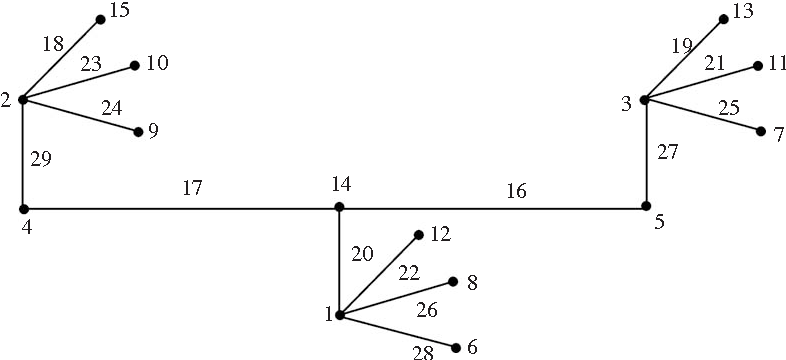•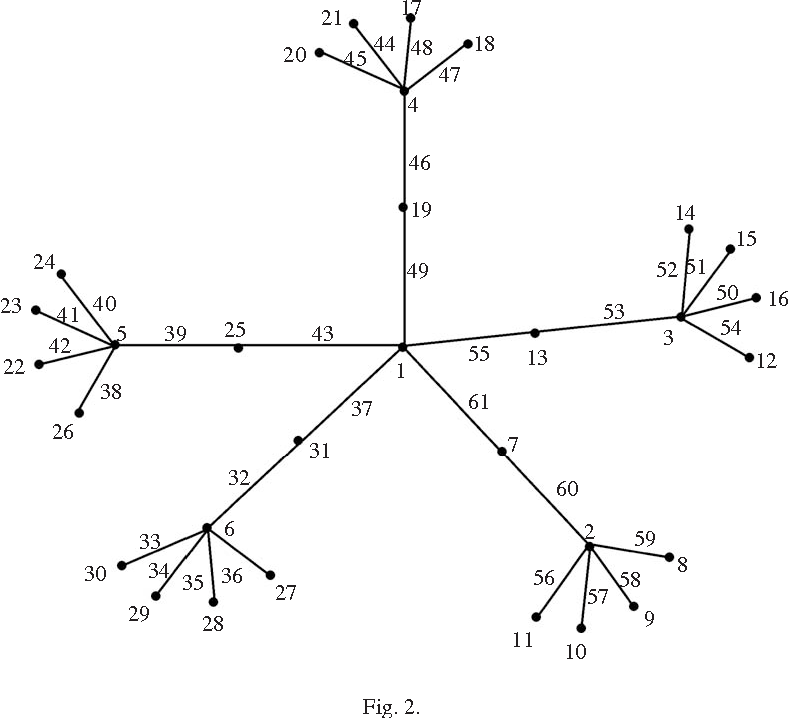•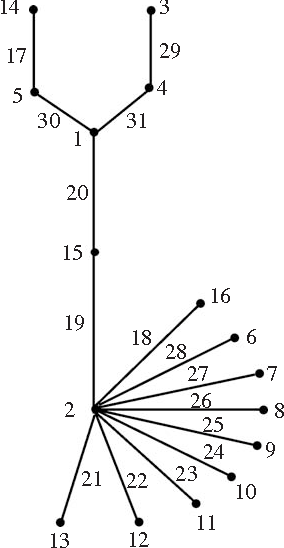•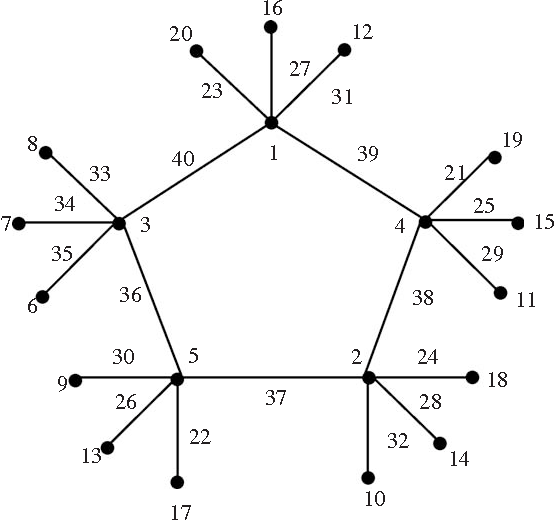•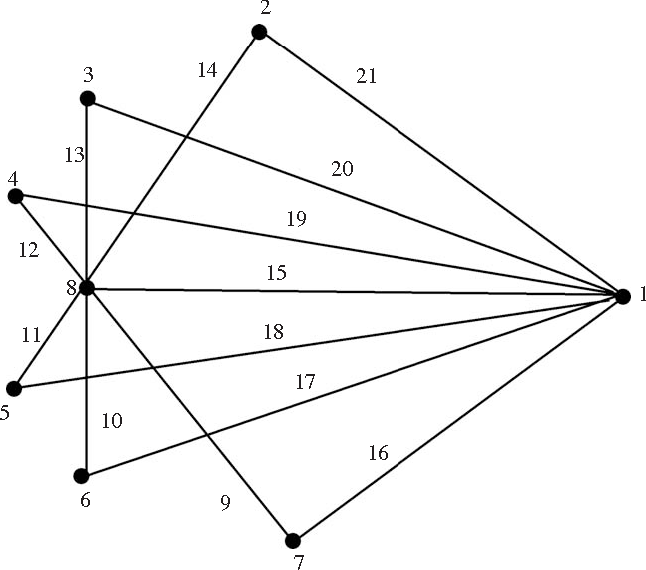2000
2000
• O. Togni
• Discret. Math.
• 2000
• Corpus ID: 205879626
The irregularity strength of a graph G is the smallest possible value of k or which we can assign positive integers not greater… Expand
•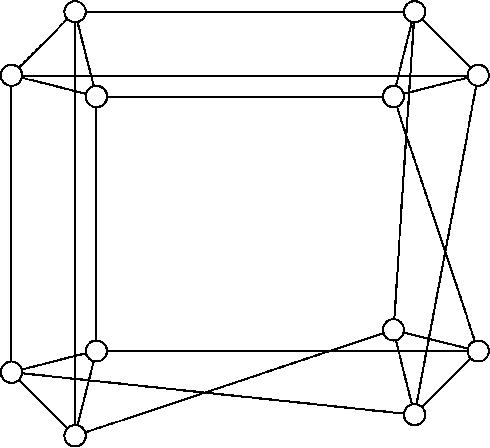•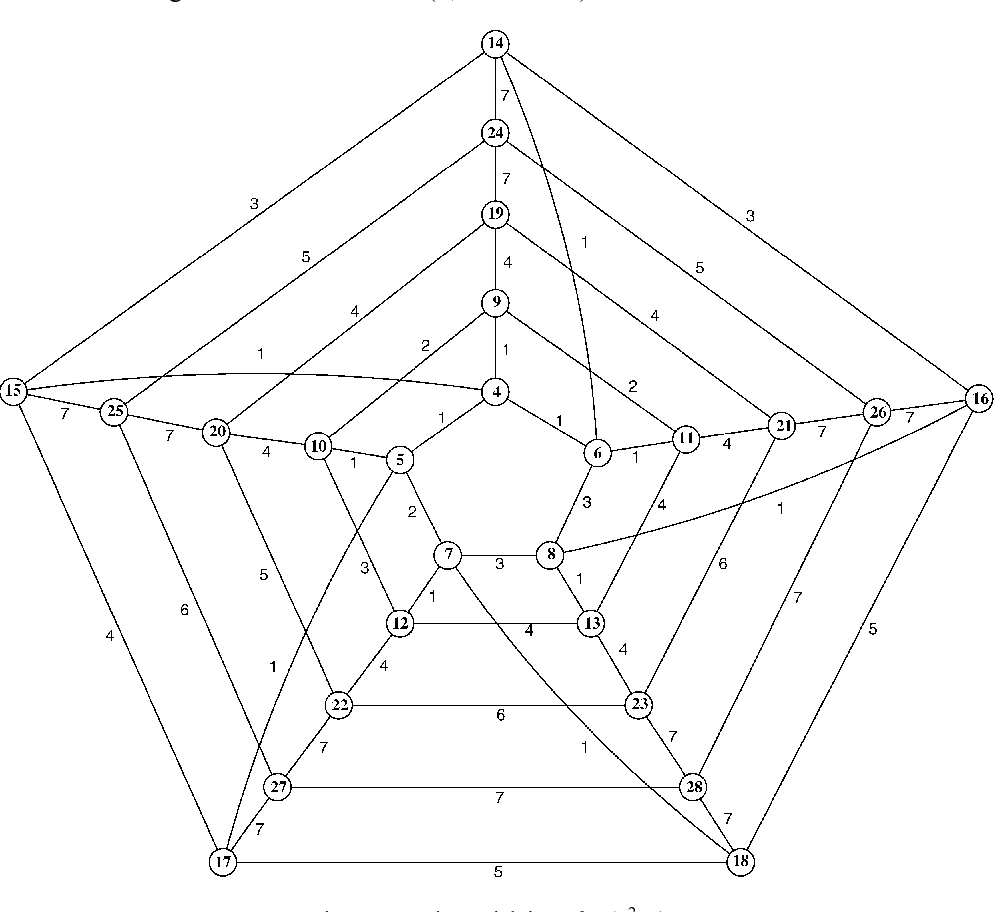1993
1993
• D. Amar
• Discret. Math.
• 1993
• Corpus ID: 34080323
Abstract As defined by Chartrand et al. , the irregularity strength of a graph G is the smallest possible value of k for which… Expand
Highly Cited
1992
Highly Cited
1992
• Discret. Appl. Math.
• 1992
• Corpus ID: 31165554
Abstract In a 1983 paper, D. Gusfield introduced a function which is called (following W.H. Cunningham, 1985) the strength of a… Expand
1991
1991
The strength of a graph is a measure of its vulnerability, strictly generalizing the edge connectivity of a weighted graph… Expand
•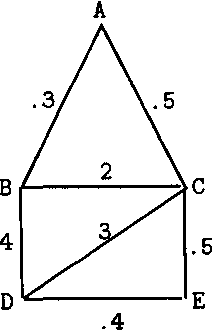Highly Cited
1989
Highly Cited
1989
• Discret. Math.
• 1989
• Corpus ID: 29102976
Abstract A network is a simple graph to which each edge has been assigned a positive integer weight. A network is irregular if… Expand